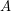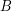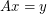# invertible

## The permanent conjecture ★★

Author(s): Kahn

Conjecture   Ifis an invertiblematrix, then there is ansubmatrixofso thatis nonzero.

Keywords: invertible; matrix; permanent

## A nowhere-zero point in a linear mapping ★★★

Author(s): Jaeger

Conjecture   Ifis a finite field with at least 4 elements andis an invertiblematrix with entries in, then there are column vectorswhich have no coordinates equal to zero such that.

Keywords: invertible; nowhere-zero flow